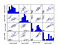# Basic Analysis of the Iris Data set Using Python

## Intro:

The Iris flower data is a multivariate data set introduced by the British statistician and biologist Ronald Fisher in his 1936 paper The use of multiple measurements in taxonomic problems as an example of linear discriminant analysis . It is sometimes called Anderson’s Iris data set because Edgar Anderson collected the data to quantify the morphologic variation of Iris flowers of three related species. Two of the three species were collected in the Gaspé Peninsula “all from the same pasture, and picked on the same day and measured at the same time by the same person with the same apparatus”.

The data set consists of 50 samples from each of three species of Iris (Iris setosa, Iris virginica and Iris versicolor). Four features were measured from each sample: the length and the width of the sepals and petals, in centimetres. Based on the combination of these four features, Fisher developed a linear discriminant model to distinguish the species from each other. (for more information ont the iris data set visit;

## Process:

`import pandasfrom pandas.tools.plotting import scatter_matriximport matplotlib.pyplot as pltfrom sklearn import model_selectionfrom sklearn.metrics import classification_reportfrom sklearn.metrics import confusion_matrixfrom sklearn.metrics import accuracy_scorefrom sklearn.linear_model import LogisticRegressionfrom sklearn.tree import DecisionTreeClassifierfrom sklearn.neighbors import KNeighborsClassifierfrom sklearn.discriminant_analysis import LinearDiscriminantAnalysisfrom sklearn.naive_bayes import GaussianNBfrom sklearn.svm import SVC`

We are using pandas to load the data. We will also use pandas next to explore the data both with descriptive statistics and data visualization.

Note that we are specifying the names of each column when loading the data. This will help later when we explore the data.

`dataset = pandas.read_csv('iris_dataset.csv')`

After loading the data via pandas, we should checkout what the content is, description andvia the following:

`dataset.head() #to check the first 10 rows of the data setdataset.tail() #to check out last 10 row of the data setdataset.describe() #to give a statistical summary about the datasetdataframe.sample(5) #pops up 5 random rows from the data set dataframe.isnull().sum() #checks out how many null info are on the dataset`

Now we visualize our data;

first with a boxplot which is going to be in the univariate form for each measurement.

`# box and whisker plotsdataset.plot(kind='box', subplots=True, layout=(2,2), sharex=False, sharey=False)plt.show()`

We can also use histogram to analysis

`# histogramsdataset.hist()plt.show()`

Now we can also look at the interactions between the variables.

First, let’s look at scatterplots of all pairs of attributes. This can be helpful to spot structured relationships between input variables.

`# scatter plot matrixscatter_matrix(dataset)plt.show()`The diagonal grouping of some pairs of attributes. This suggests a high correlation and a predictable relationship

From here we can create a validation set for our dataset:

`# Split-out validation datasetarray = dataset.valuesX = array[:,0:4]Y = array[:,4]validation_size = 0.20seed = 7X_train, X_validation, Y_train, Y_validation = model_selection.train_test_split(X, Y, test_size=validation_size, random_state=seed)`

We have splited the loaded dataset into two, 80% of which we will use to train our models and 20% that we will hold back as a validation dataset.

## Test Harness:

`# Test options and evaluation metricseed = 7scoring = 'accuracy'`

We are using the metric of ‘accuracy‘ to evaluate models. This is a ratio of the number of correctly predicted instances in divided by the total number of instances in the dataset multiplied by 100 to give a percentage (e.g. 95% accurate). We will be using the scoring variable when we run build and evaluate each model next.

## Building Models:

• Logistic Regression (LR)
• Linear Discriminant Analysis (LDA)
• K-Nearest Neighbors (KNN).
• Classification and Regression Trees (CART).
• Gaussian Naive Bayes (NB).
• Support Vector Machines (SVM).

This is a good mixture of simple linear (LR and LDA), nonlinear (KNN, CART, NB and SVM) algorithms.

`# Spot Check Algorithmsmodels = []models.append(('LR', LogisticRegression()))models.append(('LDA', LinearDiscriminantAnalysis()))models.append(('KNN', KNeighborsClassifier()))models.append(('CART', DecisionTreeClassifier()))models.append(('NB', GaussianNB()))models.append(('SVM', SVC()))# evaluate each model in turnresults = []names = []for name, model in models: kfold = model_selection.KFold(n_splits=10, random_state=seed) cv_results = model_selection.cross_val_score(model, X_train, Y_train, cv=kfold, scoring=scoring) results.append(cv_results) names.append(name) msg = "%s: %f (%f)" % (name, cv_results.mean(), cv_results.std()) print(msg)`

We will have this output:

`LR: 0.966667 (0.040825)LDA: 0.975000 (0.038188)KNN: 0.983333 (0.033333)CART: 0.975000 (0.038188)NB: 0.975000 (0.053359)SVM: 0.981667 (0.025000)`

Then we’ll choose the best algorithm: KNN seems to be the best with the value 0.983

## Make Predictions:

`# Make predictions on validation datasetknn = KNeighborsClassifier()knn.fit(X_train, Y_train)predictions = knn.predict(X_validation)print(accuracy_score(Y_validation, predictions))print(confusion_matrix(Y_validation, predictions))print(classification_report(Y_validation, predictions))`

The accuracy is 0.9 or 90%. The confusion matrix provides an indication of the three errors made. Finally, the classification report provides a breakdown of each class by precision, recall, f1-score and support showing excellent results (granted the validation dataset was small).

`#output0.9[[ 7 0 0] [ 0 11 1] [ 0 2 9]]precision recall f1-score supportIris-setosa 1.00 1.00 1.00 7Iris-versicolor 0.85 0.92 0.88 12Iris-virginica 0.90 0.82 0.86 11avg / total 0.90 0.90 0.90 30`

We have been able to analyse and make predictions with these few basic steps. Thanks for reading.

Written by

Written by

## Oluwasogo Oluwafemi Ogundowole